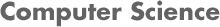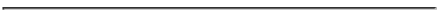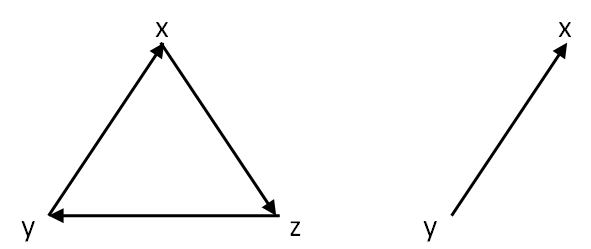### CS2223 Algorithms Homework 3 Solutions - D Term 2009

#### By PROF. CAROLINA RUIZ• Homework Problems:

1. (20 Points) Problem 1. In this problem, we observe connectivity properties of undirected and of directed graphs.

• (10 Points). Let G=(V,E) be any connected undirected graph. Prove that there is a node u in V such that removing u (together with all its incident edges) from G leaves G connected. Explain your answer in detail.
Hint: Use the tree structure constructed during the execution of BFS.

Solution: Since G is connected, then for any two nodes in G, there is a path between these two nodes in G. Pick any node s in G. Consider the tree constructed during Breadth First Search (BFS) starting at s. Now take a node x in the last level of this BFS tree. We claim the graph resulting from eliminating x from G (let's call it G-{x}) is still connected.

Let's prove our claim: Let u and v be two nodes in G-{x}. One of the following two cases holds:

• u and v appear on the same branch of the BFS tree of G. Since x was removed from the very end of a branch, then its removal cannot break the path between u and v on this tree.
• u and v appear on different branches of the BFS tree of G. Then the concatenation of the path between u and s and the path between s and v provides a path between u and v on this tree.
It is easy to see that the removal of x from G doesn't break any of the paths on the BFS tree (since x is a leaf in that tree). Hence, all the paths in the BFS tree are preserved in G-{x}, and so in any of the two cases above, u and v remain connected in G-{x}.

• (10 Points). Give an example of a graph G=(V,E) which is strongly connected (see definition in Section 3.5 of the textbook and in the textbook slides) but such that the removal of any node in G would make G not strongly connected. Note that you need to show that: (1) G is strongly connected, and that (2) for each node u in your graph, removing u and all the edges incident to u from G would result in a graph that is not strongly connected.

Solution:Strongly connected graph G (graph on the left) and G - {z} (graph on the right)

Note that G is strongly connected. That is, there for each two nodes u and v in the graph, there is a path between u and v, and a path between v and u. However, removing any node (and its incident edges) results in a graph in which there is a path from one of the remaining nodes to the other, but not vice versa. This is illustrated in the figure above for G - {z}.

2. (45 Points) Problem 2. Let G=(V,E) be an undirected graph. The complement of G is defined as the undirected graph GC = (V,EC), with the same set of nodes V, but such that an edge (u,v) belongs to EC if and only if (u,v) doesn't belong to E. That is, EC = { (u,v) | (u,v) ∉ E}.

• Adjacency Matrix Representation

1. (5 Points) Write detailed pseudo-code for an algorithm that receives a graph G=(V,E) as input, and produces GC = (V,EC) as output. Assume that both G and GC are represented using an adjacency matrix representation. The more efficient your algorithm, the better. Explain your work.

Let n be the number of nodes in G. Solution:
```For i := 1 to n {
For j:= 1 to n {
If adjacency_matrix[i,j] == 0
then adjacency_matrix[i,j] := 1
else adjacency_matrix[i,j] := 0
}
}
```
Actually, one could simplify the pseudo-code above to:
```For i := 1 to n {
For j:= 1 to n {
}
}
```

2. (10 Points) Analyze the time complexity of your algorithm above instruction by instruction. Produce a function T(n,m) that measures the runtime of your algorithm for an input graph with n nodes and m edges. Provide a tight asymptotic bound f(n,m) for T(n,m). That is, provide a function f(n,m) and prove that T(n,m) is Θ(f(n,m)).

Solution:  Time per instruction Number of iterations Total 1. For i := 1 to n { c1 n c1*n 2. For j := 1 to n { c2 n2 c2*n2 3. adjacency_matrix[i,j] := 1 - adjacency_matrix[i,j] c3 n2 c3*n2 TOTAL c1*n + c2*n2 + c3*n2 = Θ(n2)

Note that c1*n + c2*n2 + c3*n2 = Θ(n2) since for all n ≥ 1, n2 ≤ c1*n + c2*n2 + c3*n2 ≤ (c1+c2+c3)*n2.

In summary, given that all the algorithm does is to access each of the n2 cells of the adjacency matrix exactly once, the runtime of the algorithm is Θ(n2): It will have to perform at least and at most n2 operations (i.e., it is Ω(n2) and O(n2) respectively).

• Adjacency List Representation

1. (15 Points) Write another detailed pseudo-code for an algorithm that receives a graph G=(V,E) as input, and produces GC = (V,EC) as output. Assume that both G and GC are represented using an adjacency list representation. (Do NOT assume that the neighbors of a node are organized in any particular order in the node's adjacency list). The more efficient your algorithm, the better. Explain your work.

Solution: Let n be the number of nodes in G. The following algorithm to construct the adjacency list representation of GC based on that of G might not be the most efficient, but it is very easy to understand.
```
Let's use an auxiliary array neighbor of size n to record which nodes
are adjacent to the node under consideration in graph G.

For i := 1 to n {

/* initialize array neighbor: */

For j:= 1 to n {
neighbor[j] := 0
}

/* traverse the adjacency list for node i in graph G */
/* and record i's neighbors in array neighbor:       */

For each edge (i,j) incident to i in G {
neighbor[j] := 1
}

/* create the adjacency list for node i in graph GC: */

For j:= 1 to n {
If neighbor[j] == 0
then add the edge (i,j) to the adjacency list of i in GC
}

}

```

2. (15 Points) Analyze the time complexity of your algorithm above instruction by instruction. Produce a function T(n,m) that measures the runtime of your algorithm for an input graph with n nodes and m edges. Provide an upper asymptotic bound g(n,m) for T(n,m). That is, provide a function g(n,m) and prove that T(n,m) is O(g(n,m)).

Solution:  Time per instruction Number of iterations Total 1. For i := 1 to n { c1 n c1*n 2. For j:= 1 to n { c2 n2 c2*n2 3. neighbor[j] := 0 } c3 n2 c3*n2 4. For each edge (i,j) incident to i in G { c4 &Sigmani=1 degree(i) = 2m c4*2m 5. neighbor[j] := 1 } c5 &Sigmani=1 degree(i) = 2m c5*2m 6. For j:= 1 to n { c6 n2 c6*n2 7. If neighbor[j] == 0 c7 n2 c7*n2 8. then add the edge (i,j) to the adjacency list of i in GC } c8 &Sigmani=1 (n-degree(i)) = n2 - 2m c8*n2 - c8*2m TOTAL c1*n + (c2+c3+c6+c7+c8)*n2 + (c4+c5-c8)*2m = O(n2 + m) = O(n2)

Note that since m = O(n2), then O(n2 + m) = O(n2).

3. (35 Points) Problem 3. Let G=(V,E) be an undirected graph. Let u be a node in G. Recall that the degree of a node u is the number of neighbors of u in G, or in other words, the number of edges in E that are incident to u.

1. (10 Points) Write a detailed pseudo-code for an algorithm that receives a graph G=(V,E) as input with |V|=n, |E|=m, and produces an array SumNeighborsDegrees[1...n] as output, where for each node u in V, SumNeighborsDegrees[u] contains the sum of the degrees of all the neighbors of u. Assume that G is represented using an adjacency list representation. Your algorithm should run in linear time, that is it should be O(n+m).

Solution: Let n be the number of nodes in G. The following algorithm goes over the adjacency list of G twice, once calculating the degree of each node, and the second time adding together the degrees of the neighbors of each node.
```
Let's use an auxiliary array degree of size n to store
the degree of each node in G.

/* initialize array degree: */

For i:= 1 to n {
degree[i] := 0
}

/* traverse the adjacency list for each node i in graph G */
/* recording the number of i's neighbors in array degree: */

For i := 1 to n {

For each edge (i,j) incident to i in G {
degree[i] := degree[i] + 1
}
}

/* traverse the adjacency list for each node i in graph G  */
/* adding together the degrees of i's neighbors            */

For i := 1 to n {

SumNeighborsDegrees[i] := 0

For each edge (i,j) incident to i in G {
SumNeighborsDegrees[i] := SumNeighborsDegrees[i] + degree[j]
}

}

```

2. (10 Points) Prove that your algorithm above is correct.

Solution: For each u in V, we need to calculate SumNeighborsDegrees[u] which is the sum of the degrees of all the neighbors of u. Hence, we need to start by calculating the degree of each node, and then we go over the list of neighbors of each node u, adding together their degrees to determine SumNeighborsDegrees[u]. This is exactly what our algorithm above does.

3. (15 Points) Analyze the time complexity of your algorithm above instruction by instruction. Produce a function T(n,m) that measures the runtime of your algorithm for an input graph with n nodes and m edges. Prove that T(n,m) is O(n+m).

Solution:
 Time per instruction Number of iterations Total 1. For i := 1 to n { c1 n c1*n 2. degree[i] := 0 } c2 n c2*n 3. For i := 1 to n { c3 n c3*n 4. For each edge (i,j) incident to i in G { c4 &Sigmani=1 degree(i) = 2m c4*2m 5. degree[i] := degree[i] + 1 }} c5 &Sigmani=1 degree(i) = 2m c5*2m 6. For i := 1 to n { c6 n c6*n 7. SumNeighborsDegrees[i] := 0 c7 n c7*n 8. For each edge (i,j) incident to i in G { c8 &Sigmani=1 degree(i) = 2m c8*2m 9. SumNeighborsDegrees[i] := SumNeighborsDegrees[i] + degree[j]}} c9 &Sigmani=1 degree(i) = 2m c9*2m TOTAL (c1+c2+c3+c6+c7)*n + (c4+c5+c8+c9)*2m = O(n + m)